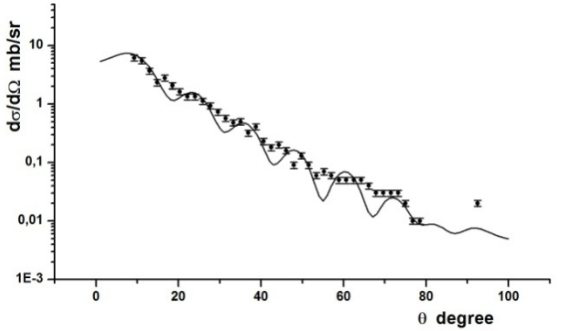# LXX International conference "NUCLEUS – 2020. Nuclear physics and elementary particle physics. Nuclear physics technologies"

Oct 11 – 17, 2020
Online
Europe/Moscow timezone

## THE ${}^{12}$C(${}^{10}$B,${}^{9}$Be)${}^{13}$N REACTION STUDY FOR ANC ${}^{13}$N→${}^{12}$C+p EVALUATION

Not scheduled
25m
Online

#### Online

Oral report Section 2. Experimental and theoretical studies of nuclear reactions.

### Speaker

S.V. Artemov (Institute of Nuclear Physics, Tashkent, Uzbekistan)

### Description

In this work the measurement of the differential cross sections (DCS) of the proton transfer reaction ${}^{12}$C(${}^{10}$B,${}^{9}$Be${}_{g.s.}$)${}^{13}$N${}_{g.s.}$. has been carried out (Fig. 1) for the ANC ${}^{13}$N${}_{g.s.}$→${}^{12}$C+p obtaining. The experiment was fulfilled at 10B ions beam of the C-200P cyclotron of the Heavy Ion Laboratory (University of Warsaw) at the energy $E_{10B}$=41.3 MeV. At this energy the proton transferring process is practically peripheral what is suggested by behavior of the testing function $R(b)$ of the MDWBA method 1 in which the single DCS calculated using the LOLA computer code . So the DCS of the reaction is expressed via the product of the ANCs squares ${(C_{10B→9Be+p})}^{2}$×${(C_{13N→12C+p})}^{2}$. The ANC ${(C_{13N→12C+p})}^{2}$ = 2.6±0.3 fm${}^{-1}$ was preliminary evaluated from the analysis (the solid curve in Fig.1), as the ANC ${(C_{10B→9Be+p})}^{2}$ is rather well known from [3,4]. The channel coupling was found to be negligible by evaluation with the FRESCO code. More correct value will be found by analysis of the data in the frameworks of the developed asymptotic reaction theory  along with a careful selection of parameters of the optical potentials.
The derived ANC for ${}^{12}$C+p→${}^{13}$N will be applied for calculation of the nuclear-astrophysical ${}^{12}$C(p,$\gamma$)${}^{13}$N reaction rates at stellar temperatures.1. Tojiboev O. R., Yarmukhamedov R., Artemov S. V., Sakuta S. B. //Phys.Rev. C94 2016, 054616.
2. R.M. DeVries, thesis, university of California, 1971; J. Perrenoud and R.M. DeVries // Phys. Lett. B36. 18, 1971.
3. Mukhamedzhanov A. M., Clark H. L., Gagliardi C. A.,.Lui Y.W., Trache L., Tribble R.E., Xu H. M. and Zhou X.G., Burjan V., Cejpek J., Kroha V., Carstoiu F. // Phys.Rev. –C56, №3. –1997. – P.1302–1312.
4. S.V. Artemov, I.R. Gulamov, E.A. Zaparov, I.Yu. Zotov, G.K. Nie. // Phys. At. Nucl. V. 59 № 3 (1996) pp. 428-438.
5. R. Yarmukhamedov, K. I. Tursunmakhatov, N. Burtebayev. // International Journal of Modern Physics: Conference Series, Vol. 49 (2019) p.1960016.

### Primary authors

S.V. Artemov (Institute of Nuclear Physics, Tashkent, Uzbekistan) N. Burtebayev (Institute of Nuclear Physics, Almaty, Kazakhstan; al-Farabi Kazakh National University, Almaty, Kazakhstan) R. Yarmukhamedov (Institute of Nuclear Physics, Tashkent, Uzbekistan) S.B. Sakuta (Russian Research Center “Kurchatov Institute”, Moscow, Russia) Marzhan Nassurlla (Institute of Nuclear Physics, Almaty, Kazakhstan; al-Farabi Kazakh National University, Almaty, Kazakhstan) N. Amandeldi (L.N.Gumilyov Eurasian National University, Nur-Sultan, Kazakhstan) B. Mauyey (L.N.Gumilyov Eurasian National University, Nur-Sultan, Kazakhstan) Maulen Nassurlla (Institute of Nuclear Physics, Almaty, Kazakhstan) F.Kh. Ergashev (Institute of Nuclear Physics, Tashkent, Uzbekistan) A.A. Karakhodzhaev (Institute of Nuclear Physics, Tashkent, Uzbekistan) O.R. Tojiboev (Institute of Nuclear Physics, Tashkent, Uzbekistan) K.I. Tursunmakhatov (Gulistan State University, Gulistan, Uzbekistan) K. Rusek (University of Warsaw, Warsaw, Poland) A. Trzcinska (University of Warsaw, Warsaw, Poland) M. Wolinska-Cichocka (University of Warsaw, Warsaw, Poland) E. Piasecki (University of Warsaw, Warsaw, Poland)

### Presentation materials

There are no materials yet.